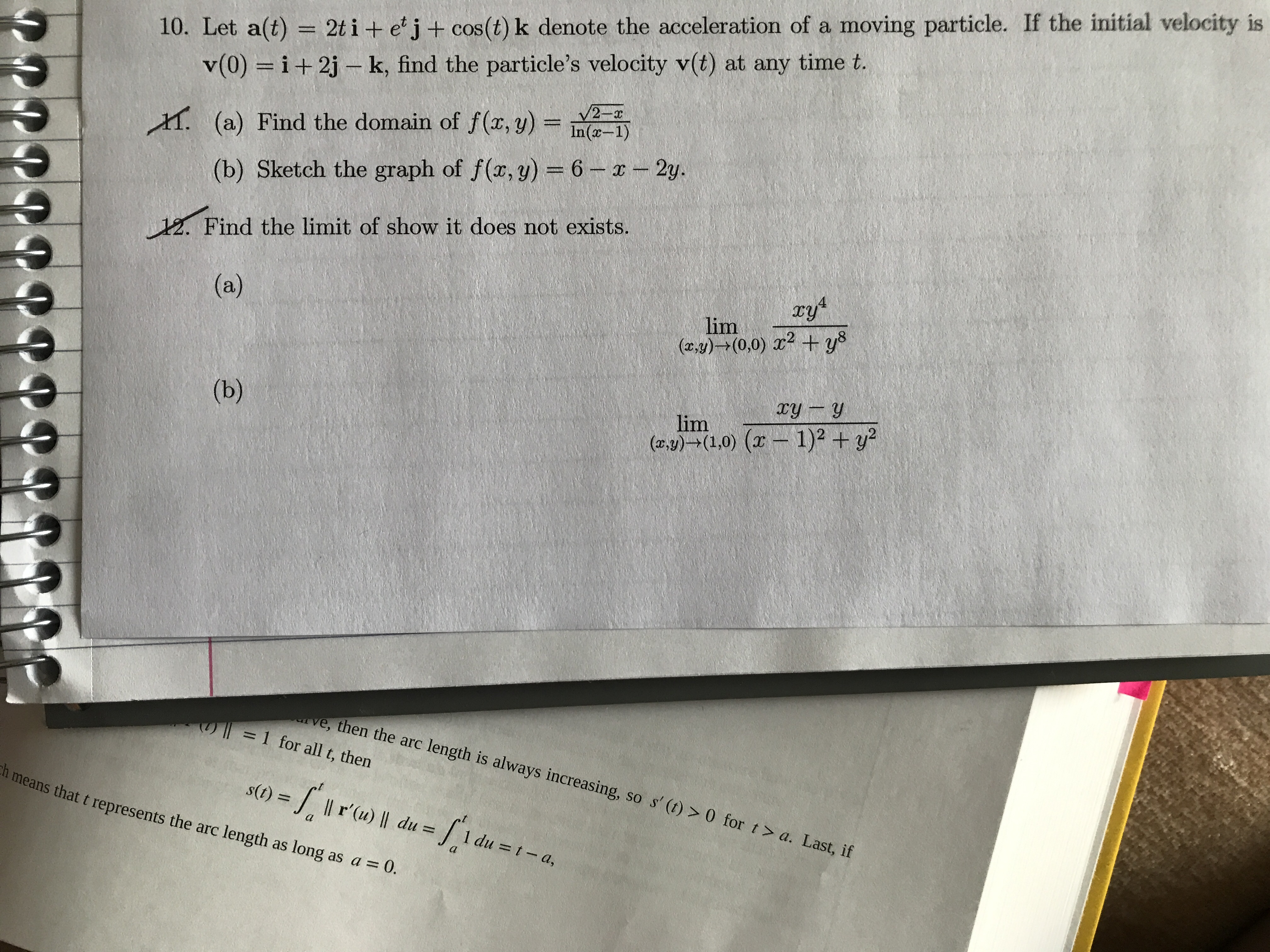# 10. Let a(t) = 2ti + e'j+ cos(t) k denote the acceleration of a moving particle. If the initial velocity is v(0) i+2j-k, find the particle's velocity v(t) at any time t. 2-x (a) Find the domain of f(x, y) = In(a-1) (b) Sketch the graph of f(r, y) 6-x2y. 12. Find the limit of show it does not exists. 4 (a) lim (ay)(0,0) 2 + ys xy y (b) lim (ay)(1,0) ( 1)2 +y2 ve, then the arc length is always increasing, so s' (t)> 0 for t> a. Last, if = 1 for all t, then s()Il r'(u) du = h means that t represents the arc length as long as a =0. -d a 1 du = t - a,

Question

Help with #10help_outlineImage Transcriptionclose10. Let a(t) = 2ti + e'j+ cos(t) k denote the acceleration of a moving particle. If the initial velocity is v(0) i+2j-k, find the particle's velocity v(t) at any time t. 2-x (a) Find the domain of f(x, y) = In(a-1) (b) Sketch the graph of f(r, y) 6-x2y. 12. Find the limit of show it does not exists. 4 (a) lim (ay)(0,0) 2 + ys xy y (b) lim (ay)(1,0) ( 1)2 +y2 ve, then the arc length is always increasing, so s' (t)> 0 for t> a. Last, if = 1 for all t, then s()Il r'(u) du = h means that t represents the arc length as long as a =0. -d a 1 du = t - a, fullscreen